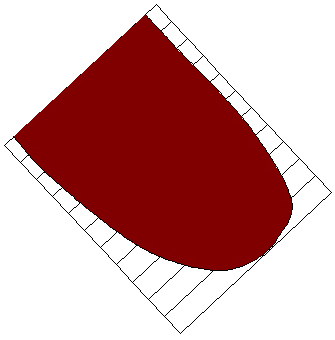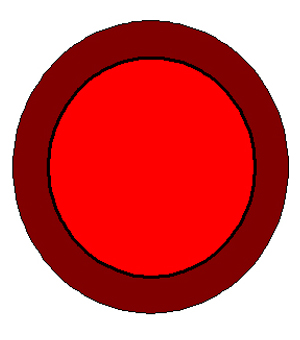##### Cardiac MRI > Pathology > Coronary Artery Disease > Simpson's Rule for Measuring Volumes

Simpson's Rule for Measuring VolumesCardiac MRI allows for accurate measurement of ventricular volume and mass due to its ability to acquire a three dimensional volume data set.

Left ventricular volumes are calculated from sequential short axis cines covering the entire ventricle. For end-diastolic volume (EDV), a tracing is made around the endocardial border at end diastole for each slice and the areas within the tracings are calculated. The end-diastolic volume of the slice is obtained from multiplying area of the end-diastolic endocardial tracing by the slice thickness of the image. The volume of each slice is then added together to obtain the overall end-diastolic volume of the left ventricle. The same procedure is performed at end-systole to calculate end-systolic volume (ESV). This method can also be used to measure myocardial mass by measuring the area of myocardium in each slice, multiplying it by the slice thickness and summing the volume of each slice. The total myocardial volume is multiplied by the density of myocardium (1.06 g/cc) to achieve myocardial mass.

Two important parameters that can be measured with cardiac MRI are Ejection fraction (EF) and Stroke Volume (SV). These values can be calculated with the following equations:

SV = EDV – ESV

EF = SV/EDVThe above diagrams demonstrate the use of Simpsons Rule. The figure on the left represents the left ventricle being imaged in the short axis plane. Slices are made from the base of the LV to the apex with each slice having a given thickness. The figure on the right shows an individual slice of the heart in the short axis view, with bright red being the ventricular space and darker red being myocardium. The area of either the ventricle or the myocardium can be calculated and multiplying this by the slice thickness gives the volume for the given slice. Adding these volumes together for all the slices gives a total volume for either the ventricle or the myocardium.